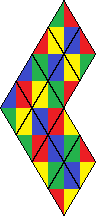# Izzi 2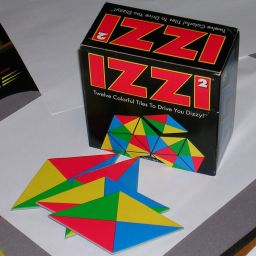Izzi 2 is a tiling puzzle made in 1993 by Binary Arts (now called ThinkFun). Like the original Izzi, it was designed by Frank Nichols. Each tile is diamond shaped, and the four edges have different colours. Every tile uses the same four colours (red, yellow, blue, green) in a different order, and every possible order is represented leading to 12 tiles (4! orderings, divided by 2 because of the 180 degree symmetry of the tile shape).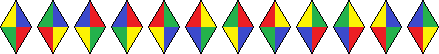The booklet coming with the puzzle shows 39 different shapes. The aim of the puzzle is to put the tiles into such a shape while making sure that all the touching tile edges match in colour.

1.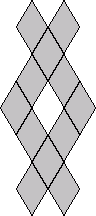2.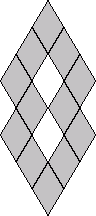3.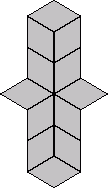4.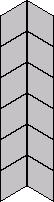5.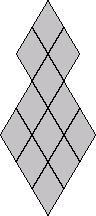6.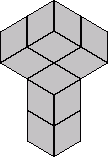7.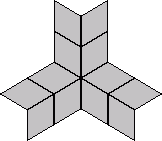8.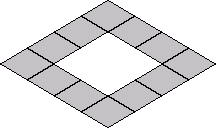9.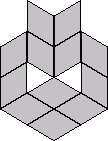10.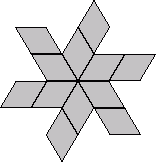11.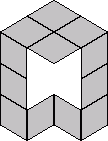12.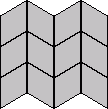13.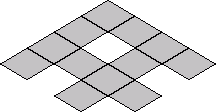14.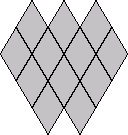15.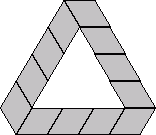16.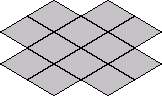17.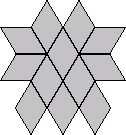18.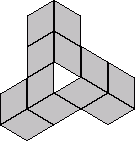19.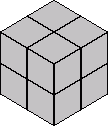20.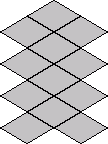21.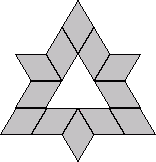22.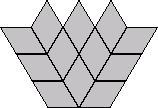23.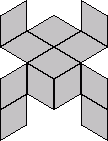24.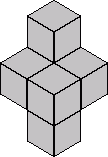25.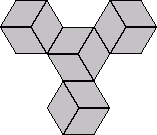26.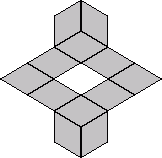27.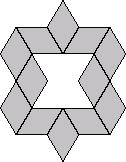28.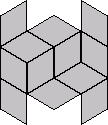29.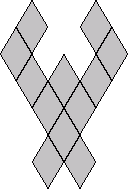30.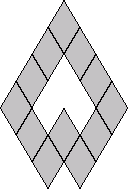31.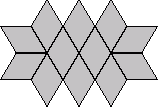32.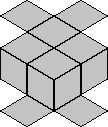33.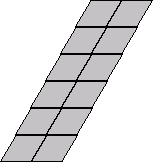34.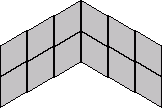35.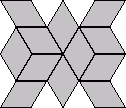36.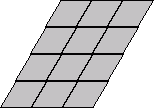37.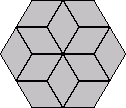38.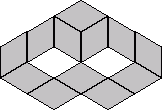39.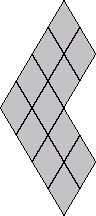## The number of positions:

Thw number of ways to place 24 tiles, each with 2 possible orientations, into a given pattern is 24!·224 = 10,409,396,852,733,332,453,861,621,760,000. This is not a valid measure of difficulty, as it does not take into account that you would not try to complete a pattern after some tiles have placed that don't match. It also is the same for all the patterns above, even though they have very different difficulty levels. A better way is to simply count the number of solutions. In a pattern with many solutions it is likely to be easier to find a solution. If the patterns are ordered from most to least solutions (i.e. approximately easiest to hardest), we get the following list:
27, 15, 10, 8, 21, 29, 13, 1, 2, 30, 23, 38, 7, 25, 11, 9, 4, 35, 34, 31, 28, 17, 20, 26, 12, 18, 33, 3, 39, 37, 32 & 22, 5, 14, 16, 36, and 24 & 19 & 6.

## Solutions:

The tiles form a complete set (i.e. every possible colour combination occurs exactly once) so if you have a solution, any permutation of the colours can be applied to create another solution. Even if you ignore such colour permutations, and any symmetry of the problem shape, all the problems have several solutions. In the table below, the colour permutation is taken into account in the number of solutions shown, but the symmetry of the shape is not.
Number of solutions Example solution Remarks 2504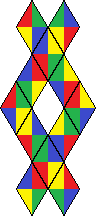2312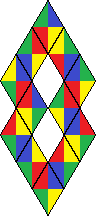160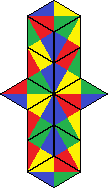520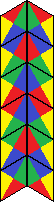32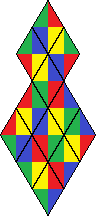8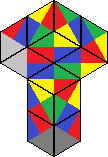Only 4 essentially different solutions, all shown here. 952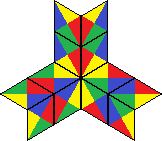3408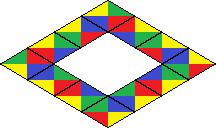652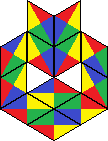4136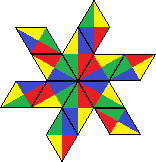928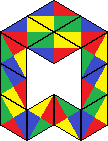198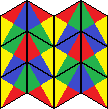2616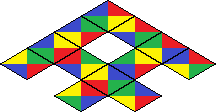26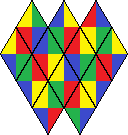4256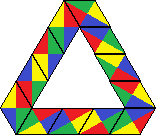24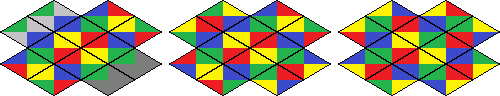Only 8 essentially different solutions, the 6 shown here, plus the last two "rotated" by 90 degrees. 272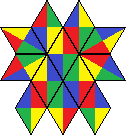196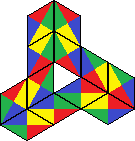8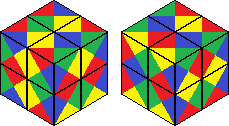Only 2 essentially different solutions, both shown here. 224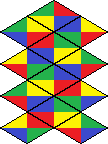3128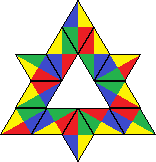52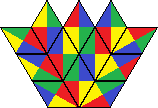1240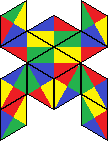8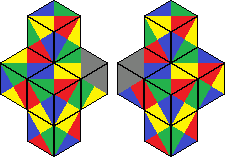Only 4 essentially different solutions, all shown here. 936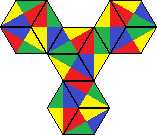204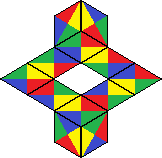6200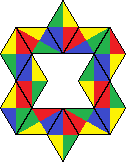348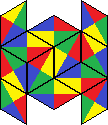2796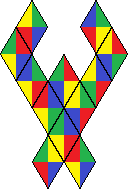2276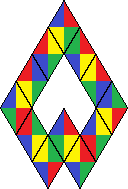448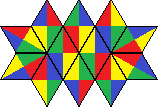52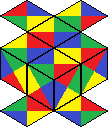176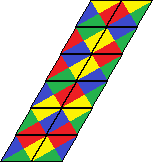482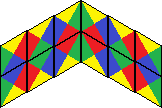484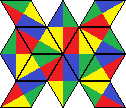16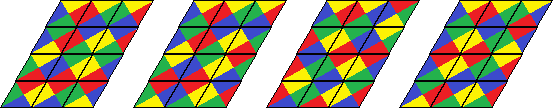Only 8 essentially different solutions, the 4 shown here, and these in "mirror image". 66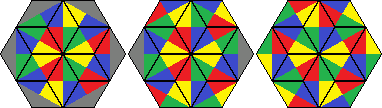Only 11 essentially different solutions, all shown here. 1178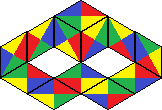124